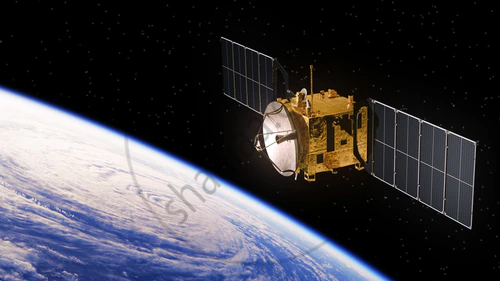# Earth Satellites

• Projection of Satellite
• Weightlessness in a Satellite
• Time Period of a Satellite
• Binding Energy of an orbiting satellite

## Notes

### Earth Satellites:

• Any object revolving around the earth.Natural Satellite

• Satellite created by nature.

• Example: - Moon is the only natural satellite of the earth.

Artificial Satellites:

• Humans built objects orbiting the earth for practical uses. There are several purposes which these satellites serve.

Example:- Practical Uses of Artificial satellites are

• Communication

• Weather observation

• Military support

• Scientific research

Determining the Time period of Earth Satellite:-

Time taken by the satellite to complete one rotation around the earth is known as the time period of the satellite.

As satellites move in circular orbits there will be a centripetal force acting on it.

"F"_c = "mv"^2/("R"_e + h) it is towards the centre.

where,

h = distance of the satellite from the earth

"F"_c = centripetal force.

"F"_G = ("GM"_e"m")/("R"_e + "h")^2

where,

"F"_g = Gravitational force

m = mass of the satellite.

"M"_e = mass of the earth

"F"_c = "F"_G

"mv"^2/("R"_e + "h")=("GM"_e"m")/("R"_e + "h")^2

"v"^2 = "GM"_e/("R"_e + "h")

"v" = sqrt("GM"_e/("R"_e +"h"))     ...(1)

This is the velocity with which satellite revolves around the earth.

The satellite covers distance = 2pi("R"_e +"h") with velocity v.

"T"=(2pi("R"_e + "h"))/"v"

(2pi("R"_e+"h"))/((sqrt"GM"_e)/("R"_e+"h"))      ...from(1)

"T"=2pi("R"_e+h)^(3/2)/sqrt"GM"_e

Special case:-

h << "R"_e (satellite is very near to the surface of the earth)

Then "T"=2pisqrt("R"_e^3/"GM"_e)

After calculating

"T" = 2pisqrt("R"_e/"g")

If you would like to contribute notes or other learning material, please submit them using the button below.

### Shaalaa.com

motion of satellites [01:09:21]
S
0%